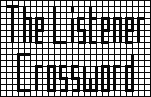## Listener Crossword 4101: Solution NotesBack to 2010 menu

## Primordial by Viking

### Puzzle solution process

It will prove useful to maintain a list of primes as they are entered, to eliminate some possibilities due to uniqueness in the grid.

When a few possibilities have to be tested, first of all sum the digits and discount any number whose sum is a multiple of 3, as that number must also be divisible by 3. Then check all possibilities for divisibility by 7 and 11. In only one case (near the end) will a larger divisor need to be found. In what follows (×3) means the number is divisible by 3, and so on.

Construct a list of 2-digit primes with odd digits:
11   13   17   19   31   37   53   59   71   73   79   97

Identify the twin (T) and mirror image (M) two-digit primes:
T: 11 / 13,   17 / 19,   71 / 73
M: 13 / 31,   17 / 71,   37 / 73,   79 / 97
The only values in common are 13, 17, 71, 73, which must be (in some order) 15a, 43a, 22d, 41d. The only T in this group is 71 / 73, which must be 15a / 41d. The only M is 17 / 71, which must be 15a / 43a. This fixes 71 and hence 17, 73 and 13. 11, 19, 31 and 37 follow from the T and M lists. From M, 79 / 97 must be 18a / 28a, so the last two entries 32a / 20d are 53 / 59, enabling the 5s to be entered.

The palindromic primes (P) promise to be useful and are quite easy to find. They have the form xyx, with x = 1, 3, 7 or 9, y = 1, 3, 5, 7 or 9, and x not equal to y (otherwise it is divisible by 111). Eliminating those with digit sum divisible by 3, we have:
131  151  191  313  353  373  737  757  797  919  959  979
The list in the clues contains 9 entries, so at most three of these are composite. A quick check reveals: 959 = 7×137, 737=11×67, 979=11×89. Hence the list must correspond to:
131   151   191   313   353   373   757   797   919
There is only one 9x9, which fixes 19d and 24a, in turn fixing the last two 2-d primes. Go through the P clue list entering reflected digits where possible.

Now look at T, noting that they must be xyz and xy(z+2) since otherwise an even digit will be used in the middle. This shows 16a starts with 1. 25d must be 311 since 315 is impossible. 30a is P, now 1x1. 31d must end in 3 and so must be one of 113 (not in xxy list), 133 (×7), 153 (×3) or 193, ie, this last value. This fixes 8d as well. 30a can’t be 151 (no 5 endings), which fixes 30a and 1a as well, both as Ps.

Now work though M, repeatedly, since some entries open up further ones. 10a (P) is 3x3 so 13d must be the last P, 7x7. 16a/23a must be 1x7, 1x9, which fixes 13d and hence 26a. 11a/11d as T means 11d ends 7. Returning to M, 9a is 9x1.

Now try the double-digit lists, D1 for xxy and D2 for xyy. D1 fixes 9a, 7d and hence 41a; D2 fixes 16a and hence 23a (T) and 17d. Also, 9d is fixed.

It is now time to look at entries with 2 of the 3 digits known.

• 38d: 333 (×3), 337 (used), 339 (×3), so it is 331.
• 44a: 311, 313 (used), 319 (×11), so it is 317.
• 6a: 913 (×11), 933 (×3), 973 (×7), 993 (×3), so it is 953, fixing 40a (M).
• 2d: 591 (×3), 597 (12a not D1), 599 (not D2), so it is 593.
• 12a: 371 (×7), 373 (not P), 377 (not D2), so it is 379, fixing 10d.
• 20a: 573 (×3), 577 (not D2), 579 (×3), so it is 571.
• 21d: 113 (used), 133 (not D2), 153 (×3), 193 (used), so it is 173, fixing last two Ps.
• 5d: 151 (not P), 153 (×3), 159 (×3), so it is 157, fixing 36d (M).
• 6d: 931 (×7), 933 (not D2), 939 (not P), so it is 937, fixing 35d (M) and 34a.
• 14a: 11d must be x37 (T), fixing 14a.
• 11a/11d: 339 (×3), 537 (×3), 737 (not P), 939 (not P), so they are 139/7.
• 32d (D2): 511 (×7), 533 (×13), 577 (used), so it is 599.
• 39a (D2): 911 (used), 933 (×3), 999 (×3), so 977, fixing 27d and a digit in 42a (M).
• 42a/33d: 973 (×7), 977/779 (not D2/D1), 979 (not P), so they are 179/971.

At this stage the grid is complete. It turns out that all primes of this form are used: a perfect grid fill. The xxy and xyy lists are, in fact, unnecessary, but eliminate much primality testing.

Back to 2010 menu